### Miscellaneous Problems 6 Statistics Class 10th Mathematics Part 1 MHB Solution

Miscellaneous Problems 6

1. The persons of O- blood group are 40%. The classification of persons based on…
2. Different expenditures incurred on the construction of a building were shown by…
3. Cumulative frequencies in a grouped frequency table are useful to find . . .…
4. The formula to find mean from a grouped frequency table is bar x = a sum f_1 ,…
5. The median of the distances covered per litre shown in the above data is in the…
6. The above data is to be shown by a frequency polygon. The coordinates of the…
7. The following table shows the income of farmers in a grape season. Find the mean…
8. The loans sanctioned by a bank for construction of farm ponds are shown in the…
9. The weekly wages of 120 workers in a factory are shown in the following…
10. The following frequency distribution table shows the amount of aid given to 50…
11. The distances covered by 250 public transport buses in a day is shown in the…
12. The prices of different articles and demand for them is shown in the following…
13. The following frequency table shows the demand for a sweet and the number of…
14. Draw a histogram for the following frequency distribution.
15. In a handloom factory different workers take different periods of time to weave…
16. The time required for students to do a science experiment and the number of…
17. Draw a frequency polygon for the following grouped frequency distribution…
18. The following table shows the average rainfall in 150 towns. Show the…
19. Observe the adjacent pie diagram. It shows the percentages of number of…
20. The following table shows causes of noise pollution. Show it by a pie diagram.…
21. A survey of students was made to know which game they like. The data obtained…
22. Medical check up of 180 women was conducted in a health centre in a village. 50…
23. On an environment day, students in a school planted 120 trees under plantation…

###### Miscellaneous Problems 6
Question 1.

Find the correct answer from the alternatives given.

The persons of O- blood group are 40%. The classification of persons based on blood groups is to be shown by a pie diagram. What should be the measures of angle for the persons of O- blood group?
A. 114°

B. 140°

C. 104°

D. 144°

Given is, percentage of persons of O- blood group = 40%

⇒ Sample of persons of O- blood group = 40

& Total sample of persons = 100

(∵, 40/100 implies that out of 100 samples, 40 are persons of O- blood group)

And we know,⇒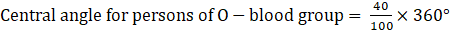⇒ Central angle for persons of O- blood group = 144°

Thus, the correct option is (D).

Question 2.

Find the correct answer from the alternatives given.

Different expenditures incurred on the construction of a building were shown by a pie diagram. The expenditure
Rs 45,000 on cement was shown by a sector of central angle of 75°. What was the total expenditure of the construction?
A. 2,16,000

B. 3,60,000

C. 4,50,000

D. 7,50,000

The pie diagram shows, the central angle for expenditure on cement = 75°

Also, expenditure on cement = Rs. 45,000

We know that,⇒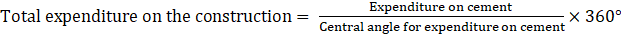⇒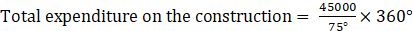⇒ Total expenditure on the construction = 216000

Thus, the correct option is (A).

Question 3.

Find the correct answer from the alternatives given.

Cumulative frequencies in a grouped frequency table are useful to find . . .
A. Mean

B. Median

C. Mode

D. All of these

The cumulative frequency of a set of data or class intervals of a frequency table is the sum of the frequencies of the data up to a required level. It can be used to determine the number of items that have values below a particular level.

Mean is simple or arithmetic average of a range of values or quantities, computed by dividing the total of all values by the number of values. And The mode of a set of data values is the value that appears most often.

While, median is the middle value of the set of ordered data. The position of the median is given by {(n + 1)/2}th value, where n is the number of values in a set of data.

So, the formula can be applied in the cumulative frequency in an arranged data to find the median.

Thus, (B) is the correct option.

Question 4.

Find the correct answer from the alternatives given.

The formula to find mean from a grouped frequency table is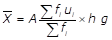In the formula ui =……
A.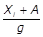B.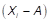C.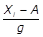D.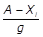Among the given option, (Xi – A)/g is the correct option.

Where Xi = values in the given data corresponding to ith position.

A = Assumed mean

g = class size

Thus, (C) is the correct option.

Question 5.

Find the correct answer from the alternatives given.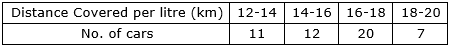The median of the distances covered per litre shown in the above data is in the group . . . . .
A. 12-14

B. 14-16

C. 16-18

D. 18-20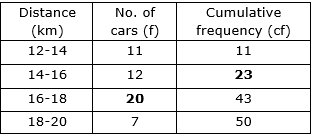Here, (n/2)th = (50/2)th = 25th term

cf = 23<25 ⇒ Median class = 16-18

Median class is the next class of interval of cumulative frequency.

Thus, option (C) is correct.

Question 6.

Find the correct answer from the alternatives given.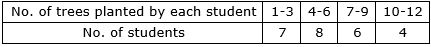The above data is to be shown by a frequency polygon. The coordinates of the points to show number of students in the class 4-6 are . . . .
A. (4, 8)

B. (3, 5)

C. (5, 8)

D. (8, 4)

First, draw a table converting the given class into continuous class.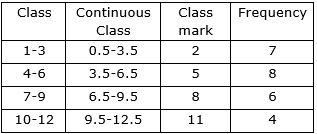Drawing it into a frequency polygon.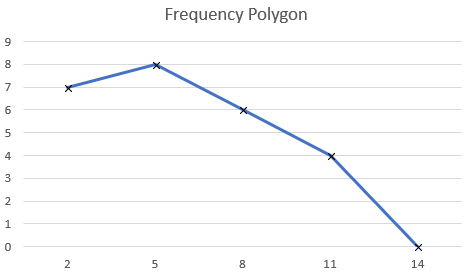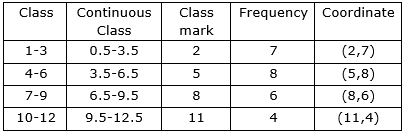So, the coordinates of the points to show number of students in the class 4-6 are (5,8).

Thus, option (C) is correct.

Question 7.

The following table shows the income of farmers in a grape season. Find the mean of their income.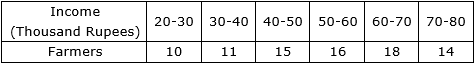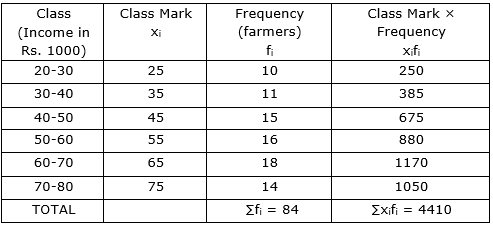Mean is given by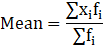⇒ Mean = 4410/84

⇒ Mean = 52.5

Now, since the income is given in thousand rupees.

Then, Mean = 52.5 × 1000

⇒ Mean = 52500

Thus, mean income is Rs. 52,500.

Question 8.

The loans sanctioned by a bank for construction of farm ponds are shown in the following table. Find the mean of the loans.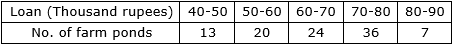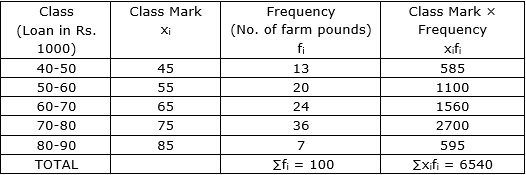Mean is given by⇒ Mean = 6540/100

⇒ Mean = 65.4

Now, since the loan is given in thousand rupees.

Then, Mean = 65.4 × 1000

⇒ Mean = 65400

Thus, mean loan is Rs. 65,400.

Question 9.

The weekly wages of 120 workers in a factory are shown in the following frequency distribution table. Find the mean of the weekly wages.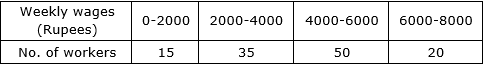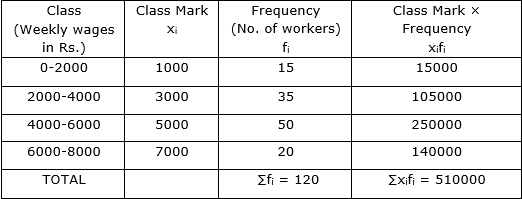Mean is given by⇒ Mean = 510000/120

⇒ Mean = 4250

Thus, mean weekly wages is Rs. 4250.

Question 10.

The following frequency distribution table shows the amount of aid given to 50 flood affected families. Find the mean of the amount of aid.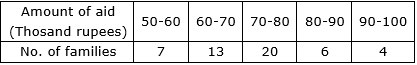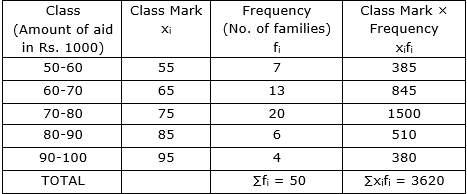Mean is given by⇒ Mean = 3620/50

⇒ Mean = 72.4

Now, since the amount of aid is given in thousand rupees.

Then, Mean = 72.4 × 1000

⇒ Mean = 72400

Thus, mean amount of aid is Rs. 72,400.

Question 11.

The distances covered by 250 public transport buses in a day is shown in the following frequency distribution table. Find the median of the distances.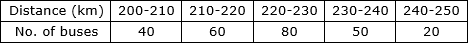Let us prepare cumulative frequency table: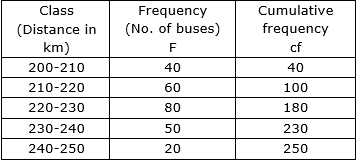Here, N = 250

N/2 = 250/2 = 125

Since, cumulative frequency 180 is just greater than 125. ⇒ median class = 220-230

Median is given by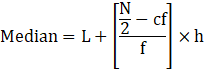Where l = lower limit of the median class

N = Sum of frequencies

h = class interval of the median class

cf = Cumulative frequency of the class preceding the median class

f = Frequency of the median class

Here, Median class = 220-230

L = 220

N/2 = 125

h = 10

cf = 100

f = 80

Putting all these values in the median formula, we get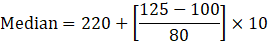⇒ Median = 220 + 250/80

⇒ Median = 220 + 3.125 = 223.125

Or Median is approximately 223.13 km

Thus, median of the distances is 223.13 km.

Question 12.

The prices of different articles and demand for them is shown in the following frequency distribution table. Find the median of the prices.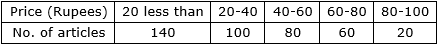Let us prepare cumulative frequency table: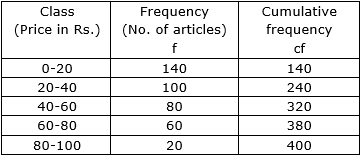Here, N = 400

N/2 = 400/2 = 200

Since, cumulative frequency 240 is just greater than 200 ⇒ median class = 20-40

Median is given byWhere l = lower limit of the median class

N = Sum of frequencies

h = class interval of the median class

cf = Cumulative frequency of the class preceding the median class

f = Frequency of the median class

Here, Median class = 20-40

L = 20

N/2 = 200

h = 20

cf = 140

f = 100

Putting all these values in the median formula, we get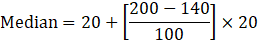⇒ Median = 20 + 1200/100

⇒ Median = 20 + 12 = 32

Thus, median of the prices is Rs. 32.

Question 13.

The following frequency table shows the demand for a sweet and the number of customers. Find the mode of demand of sweet.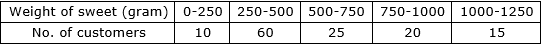Given the distribution table, notice that maximum number of customers has demand for 250-500 grams. (Since, 60 is the maximum number of customers)

So, modal class = 250-500

Mode is given by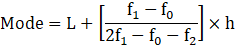Where L = lower class limit of the modal class

h = class interval of the modal class

f1 = frequency of the modal class

f0 = frequency of the class preceding the modal class

f2 = frequency of the class succeeding the modal class

Here, Modal class = 250-500

L = 250

h = 250

f1 = 60

f0 = 10

f2 = 25

Putting these values in the formula,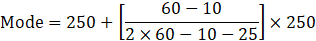⇒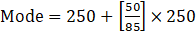⇒ Mode = 250 + 147.06

⇒ Mode = 397.06

Thus, mode of demand of sweet is 397.06 grams.

Question 14.

Draw a histogram for the following frequency distribution.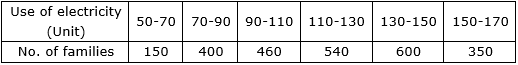Let values in use of electricity be x-values and values in no. of families be y-axis.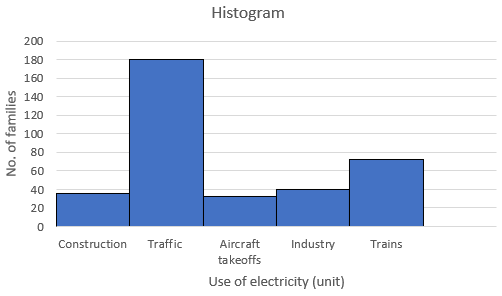Thus, this is the histogram.

Question 15.

In a handloom factory different workers take different periods of time to weave a saree. The number of workers and their required periods are given below. Present the information by a frequency polygon.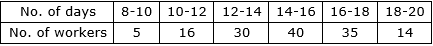First, draw a frequency table.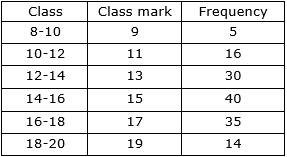Drawing it into a frequency polygon.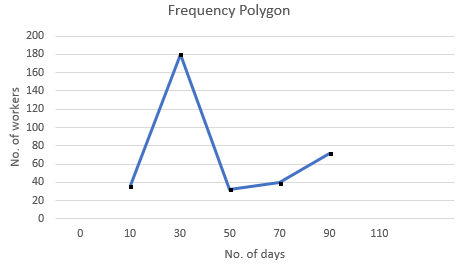Question 16.

The time required for students to do a science experiment and the number of students is shown in the following grouped frequency distribution table. Show the information by a histogram and also by a frequency polygon.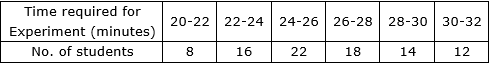First, draw a frequency table.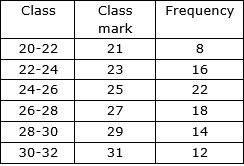Drawing it on a histogram.

Let time required for experiment (minutes) be x-values and number of students be y-axis.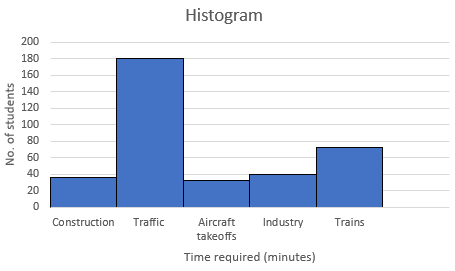Drawing it into a frequency polygon.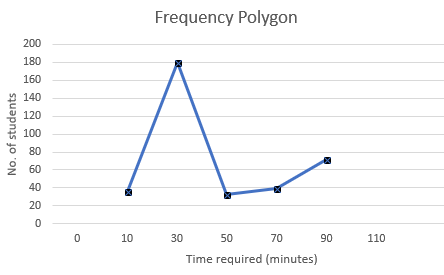Question 17.

Draw a frequency polygon for the following grouped frequency distribution table.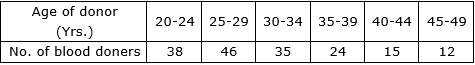First, draw a frequency table.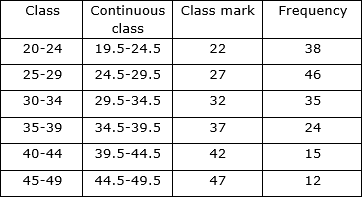The representation into a frequency polygon will be as such.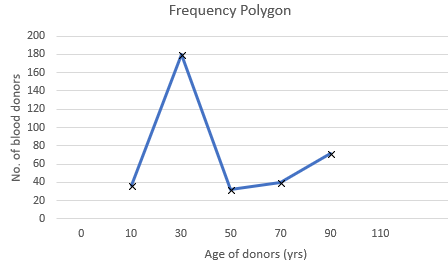Question 18.

The following table shows the average rainfall in 150 towns. Show the information by a frequency polygon.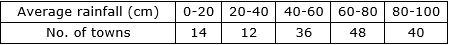First, draw a frequency table.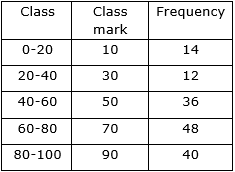Representing the information into a frequency polygon.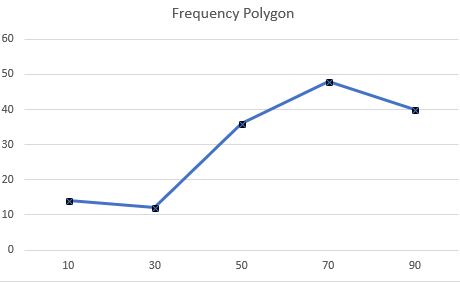Question 19.

Observe the adjacent pie diagram. It shows the percentages of number of vehicles passing a signal in a town between 8 am and 10 am

(1) Find the central angle for each type of vehicle.

(2) If the number of two-wheelers is 1200, find the number of all vehicles.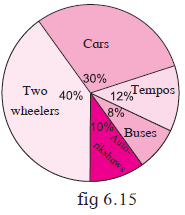(1) To find central angle for cars:

Given that, percentage of cars passing a signal in a town = 30%

⇒ Number of cars = 30

& Total number of vehicles = 100

Central angle for cars is given by,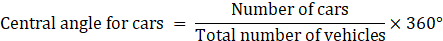⇒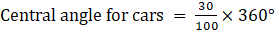⇒ Central angle for cars = 108°

Thus, central angle for cars is 108°.

To find central angle for tempos:

Given that, percentage of tempos passing a signal in a town = 12%

⇒ Number of tempos = 12

& Total number of vehicles = 100

Central angle for tempos is given by,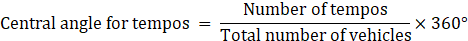⇒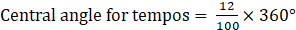⇒ Central angle for tempos = 43.2°

By approximating, we get

Central angle for tempos = 43°

Thus, central angle for tempos is 43.2°.

To find central angle for buses:

Given that, percentage of buses passing a signal in a town = 8%

⇒ Number of buses = 8

& Total number of vehicles = 100

Central angle for buses is given by,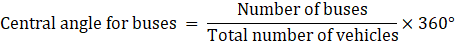⇒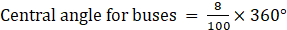⇒ Central angle for buses = 28.8°

By approximating, we get

Central angle for buses = 29°

Thus, central angle for buses is 29°.

To find central angle for auto-rikshaws:

Given that, percentage of auto-rikshaws passing a signal in a town = 10%

⇒ Number of auto-rikshaws = 10

& Total number of vehicles = 100

Central angle for auto-rikshaws is given by,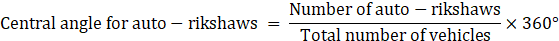⇒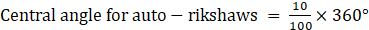⇒ Central angle for auto-rikshaws = 36°

Thus, central angle for auto-rikshaws is 36°.

To find central angle for two-wheelers:

Given that, percentage of two-wheelers passing a signal in a town = 40%

⇒ Number of two-wheelers = 40

& Total number of vehicles = 100

Central angle for two-wheelers is given by,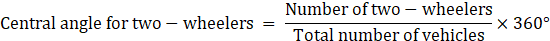⇒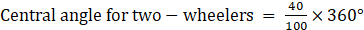⇒ Central angle for two-wheelers = 144°

Thus, central angle for two-wheelers is 144°.

Check: Add all central angles for vehicles (cars + tempos + buses + auto-rikshaws + two-wheelers) = 108° + 43° + 29° + 36° + 144° = 360°

Hence, it’s correct.

(2). Given: Number of two-vehicles = 1200

And central angle for two-vehicles = 144° (as found in part (1))

Then,⇒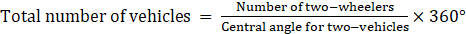⇒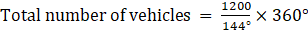⇒ Total number of vehicles = 3000

Thus, there are total 3000 vehicles in all.

Question 20.

The following table shows causes of noise pollution. Show it by a pie diagram.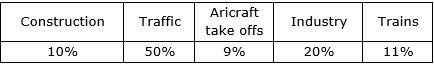Let us find the measures of central angles and show them in a table.

Know that,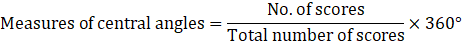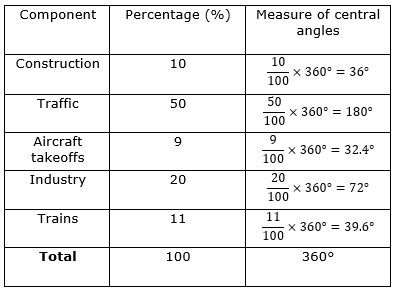Now we shall show the table into a pie chart.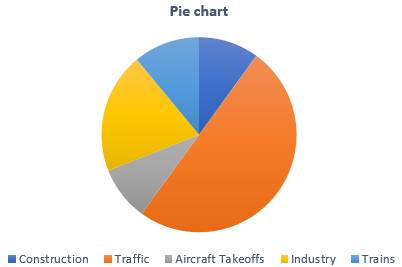Question 21.

A survey of students was made to know which game they like. The data obtained in the survey is presented in the adjacent pie diagram. If the total number of students are 1000,

(1) How many students like cricket?

(2) How many students like football?

(3) How many students prefer other games?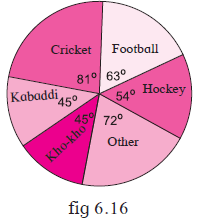(1) Central angle for cricket = 81°

Total number of students = 1000

Then,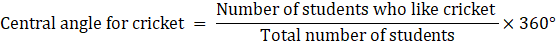⇒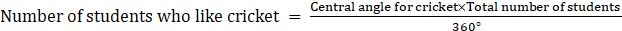⇒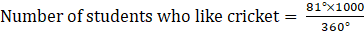⇒ Number of students who like cricket = 225

Thus, 225 students like cricket.

(2) Central angle for football = 63°

Total number of students = 1000

Then,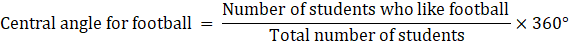⇒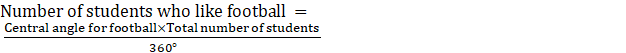⇒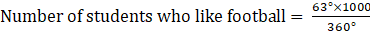⇒ Number of students who like football = 175

Thus, 175 students like football.

(3) Central angle for other games = 72°

Total number of students = 1000

Then,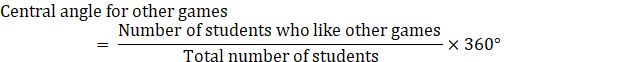⇒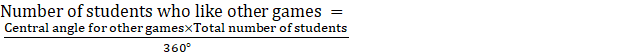⇒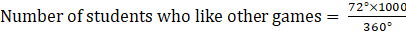⇒ Number of students who like other games = 200

Thus, 200 students like other games.

Question 22.

Medical check up of 180 women was conducted in a health centre in a village. 50 of them were short of haemoglobin, 10 suffered from cataract and 25 had respiratory disorders. The remaining women were healthy. Show the information by a pie diagram.

First lets show it by a table.

Know that,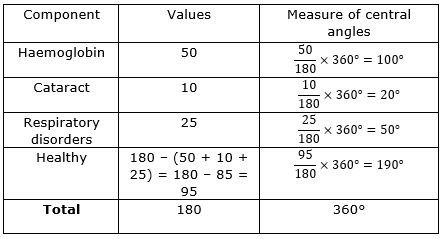Now we shall show the table into a pie chart.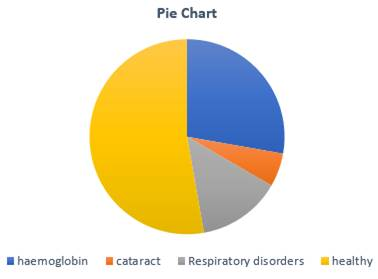Question 23.

On an environment day, students in a school planted 120 trees under plantation project. The information regarding the project is shown in the following table. Show it by a pie diagram.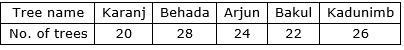First lets show it by a table.

Know that,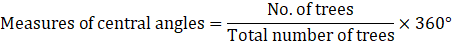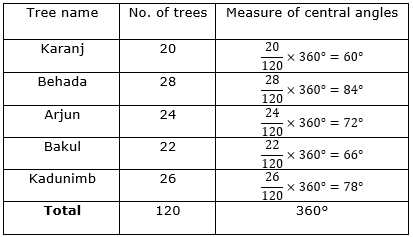Now we shall show the table into a pie chart.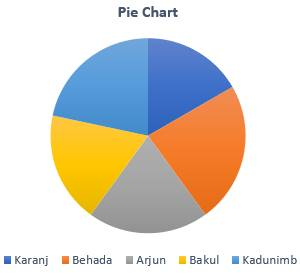## PDF FILE TO YOUR EMAIL IMMEDIATELY PURCHASE NOTES & PAPER SOLUTION. @ Rs. 50/- each (GST extra)

SUBJECTS

HINDI ENTIRE PAPER SOLUTION

MARATHI PAPER SOLUTION
SSC MATHS I PAPER SOLUTION
SSC MATHS II PAPER SOLUTION
SSC SCIENCE I PAPER SOLUTION
SSC SCIENCE II PAPER SOLUTION
SSC ENGLISH PAPER SOLUTION
SSC & HSC ENGLISH WRITING SKILL
HSC ACCOUNTS NOTES
HSC OCM NOTES
HSC ECONOMICS NOTES
HSC SECRETARIAL PRACTICE NOTES

2019 Board Paper Solution

HSC ENGLISH SET A 2019 21st February, 2019

HSC ENGLISH SET B 2019 21st February, 2019

HSC ENGLISH SET C 2019 21st February, 2019

HSC ENGLISH SET D 2019 21st February, 2019

SECRETARIAL PRACTICE (S.P) 2019 25th February, 2019

HSC XII PHYSICS 2019 25th February, 2019

CHEMISTRY XII HSC SOLUTION 27th, February, 2019

OCM PAPER SOLUTION 2019 27th, February, 2019

HSC MATHS PAPER SOLUTION COMMERCE, 2nd March, 2019

HSC MATHS PAPER SOLUTION SCIENCE 2nd, March, 2019

SSC ENGLISH STD 10 5TH MARCH, 2019.

HSC XII ACCOUNTS 2019 6th March, 2019

HSC XII BIOLOGY 2019 6TH March, 2019

HSC XII ECONOMICS 9Th March 2019

SSC Maths I March 2019 Solution 10th Standard11th, March, 2019

SSC MATHS II MARCH 2019 SOLUTION 10TH STD.13th March, 2019

SSC SCIENCE I MARCH 2019 SOLUTION 10TH STD. 15th March, 2019.

SSC SCIENCE II MARCH 2019 SOLUTION 10TH STD. 18th March, 2019.

SSC SOCIAL SCIENCE I MARCH 2019 SOLUTION20th March, 2019

SSC SOCIAL SCIENCE II MARCH 2019 SOLUTION, 22nd March, 2019

XII CBSE - BOARD - MARCH - 2019 ENGLISH - QP + SOLUTIONS, 2nd March, 2019

HSC Maharashtra Board Papers 2020

(Std 12th English Medium)

HSC ECONOMICS MARCH 2020

HSC OCM MARCH 2020

HSC ACCOUNTS MARCH 2020

HSC S.P. MARCH 2020

HSC ENGLISH MARCH 2020

HSC HINDI MARCH 2020

HSC MARATHI MARCH 2020

HSC MATHS MARCH 2020

SSC Maharashtra Board Papers 2020

(Std 10th English Medium)

English MARCH 2020

HindI MARCH 2020

Hindi (Composite) MARCH 2020

Marathi MARCH 2020

Mathematics (Paper 1) MARCH 2020

Mathematics (Paper 2) MARCH 2020

Sanskrit MARCH 2020

Important-formula

THANKS